# Mahler method

(diff) ← Older revision | Latest revision (diff) | Newer revision → (diff)

In 1929, K. Mahler [a1] started the study of transcendence properties of the values of analytic functionssatisfying certain functional equations. A simple example is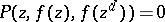, whereis a polynomial with algebraic coefficients and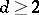an integer. For instance, the function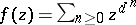, which is analytic in the unit disc of(cf. also Analytic function), satisfies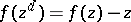, and Mahler proved that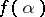is transcendental (cf. also Transcendental number) wheneveris an algebraic number satisfying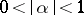.

The functional equation is used to derive many points from the starting one (in the previous example the points are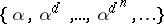), and this iteration yields points close to the origin.

Mahler's proof involves the construction of an auxiliary polynomial. This construction is different from Hermite's one, since the polynomial is not explicit, and also different from Siegel's, Gel'fond's or Schneider's ones (cf. also Gel'fond–Schneider method; Schneider method), since it rests on an argument of linear algebra rather than on the Thue–Siegel lemma (cf. also Dirichlet principle): No bound for the height of the coefficients is required.

Mahler also worked with functions of several variables [a1], [a2], introducing transformationsgiven bymonomials. By this method he even obtained results of algebraic independence [a3].

The topic was somehow forgotten until 1969 [a4]. Thanks to the work of several mathematicians, including J.H. Loxton, A.J. van der Poorten, K.K. Kubota, K. Nishioka, P.G. Becker, M. Amou, and T. Töpfer (see [a5]), general results are now available for the transcendence and algebraic independence of values of such functions, in one or several variables. This method turns out to be one of the most efficient ones for proving strong results of algebraic independence. Here is an example.

Let,be positive integers withandan algebraic number with. For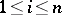, let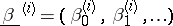be a sequence of algebraic numbers satisfying a linear recurrence relation. Assume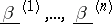are linearly independent. Then thenumbersare algebraically independent.

Also, sharp estimates of Diophantine approximations (transcendence measures as well as measures of algebraic independence) have been obtained. A far-reaching extension of Mahler's vanishing theorem was given by D.W. Masser in 1982.

Mahler's early paper [a1] contains the transcendence of the Thue–Morse number, whose binary expansionis given by the fixed point starting withof the substitution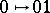and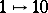(the related functional equation is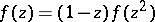). More generally, Mahler's method has interesting deep connections with automata theory (cf. also Formal languages and automata). It is conjectured that a number whose expansion in an integral basis is given by an automaton is either rational or else transcendental.

One of Mahler's goals (see [a4]) was to derive from his method the transcendence of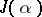for algebraicwith. Here,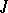is the modular function, which satisfies indeed functional equations, namely the modular equations relating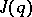andfor any. This conjecture was proved only in 1995 (see Gel'fond–Schneider method).

How to Cite This Entry:
Mahler method. Encyclopedia of Mathematics. URL: http://encyclopediaofmath.org/index.php?title=Mahler_method&oldid=16978
This article was adapted from an original article by Michel Waldschmidt (originator), which appeared in Encyclopedia of Mathematics - ISBN 1402006098. See original article